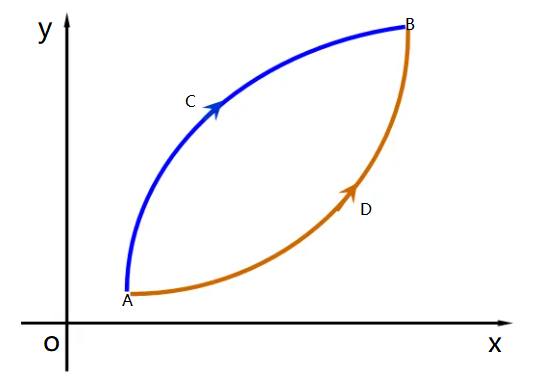安卓手机扫描二维码安装App﻿$f(x)$﻿ 在区间 ﻿$I$﻿ 上连续，如果对I上任意两点 ﻿$x_1,x_2$﻿ ，恒有

﻿$f \Big ( \dfrac{x_1+x_2}{2} \Big )$﻿﻿$<$﻿ ﻿$\dfrac{f(x_1)+f(x_2)}{2}$﻿

﻿$f \Big ( \dfrac{x_1+x_2}{2} \Big )$﻿ ﻿$>$﻿ ﻿$\dfrac{f(x_1)+f(x_2)}{2}$﻿

A.  ﻿$f'(x)>0$﻿ 时函数是增函数，﻿$f''(x)>0$﻿ 时函数图形是凹的

B. ﻿$f''(x)>0$﻿ 时函数是增函数，﻿$f''(x)>0$﻿ 时函数图形是凸的

C. ﻿$f'(x)<0$﻿ 时函数是减函数，﻿$f''(x)<0$﻿ 时函数图形是凹的

D. ﻿$f''(x)<0$﻿ 时函数是减函数，﻿$f''(x)<0$﻿ 时函数图形是凸的苹果手机扫描二维码安装App